# How to conditionally format dates and time in Excel - built-in rules and formulas

If you are a regular visitor of this blog, you've probably noticed a few recent articles covering different aspects of Excel conditional formatting. Those tutorials explain how to apply Excel's built-in rules and make your own conditional formats based on formulas.

And now we will leverage this knowledge and create spreadsheets that differentiate between weekdays and weekends, highlight public holidays and display a coming deadline or delay. In other words, we are going to apply Excel conditional formatting to dates.

If you have some basic knowledge of Excel formulas, then you are most likely familiar with some of date and time functions such as NOW, TODAY, DATE, WEEKDAY, etc. In this tutorial, we are going to take this functionality a step further to conditionally format Excel dates in the way you want.

## Excel conditional formatting for dates (built-in rules)

Microsoft Excel provides 10 options to format selected cells based on the current date.

1. To apply the formatting, you simply go to the Home tab > Conditional Formatting > Highlight Cell Rules and select A Date Occurring.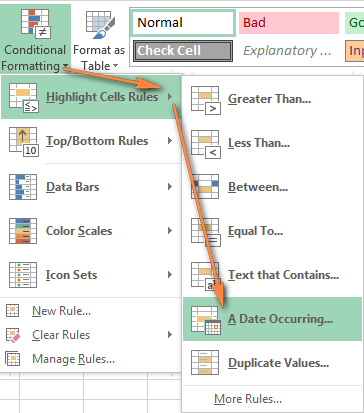2. Select one of the date options from the drop-down list in the left-hand part of the window, ranging from last month to next month.
3. Finally, choose one of the pre-defined formats or set up your custom format by choosing different options on the Font, Border and Fill tabs. If the Excel standard palette does not suffice, you can always click the More colors… button.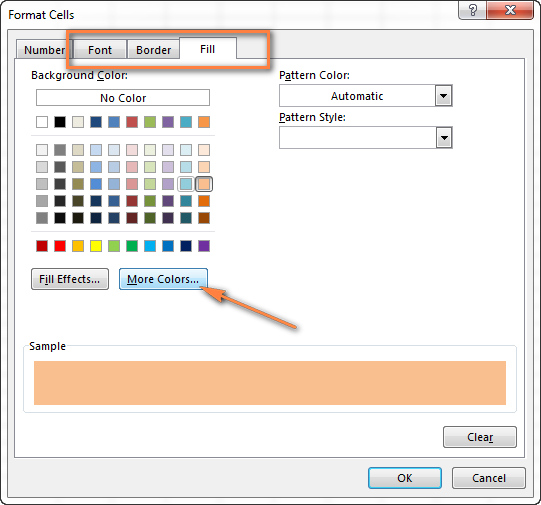4. Click OK and enjoy the result! : )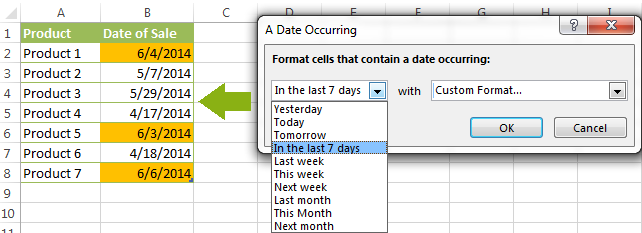However, this fast and straightforward way has two significant limitations - 1) it works for selected cells only and 2) the conditional format is always applied based on the current date.

## Excel conditional formatting formulas for dates

If you want to highlight cells or entire rows based on a date in another cell, or create rules for greater time intervals (i.e. more than a month from the current date), you will have to create your own conditional formatting rule based on a formula. Below you will find a few examples of my favorite Excel conditional formats for dates.

### How to highlight weekends in Excel

Regrettably, Microsoft Excel does not have a built-in calendar similar to Outlook's. Well, let's see how you can create your own automated calendar with quite little effort.

When designing your Excel calendar, you can use the =DATE(year,month,date) function to display the days of the week. Simply enter the year and the month's number somewhere in your spreadsheet and reference those cells in the formula. Of course, you could type the numbers directly in the formula, but this is not a very efficient approach because you would have to adjust the formula for each month.

The screenshot below demonstrates the DATE function in action. I used the formula `=DATE(\$B\$2,\$B\$1,B\$4)` which is copied across row 5.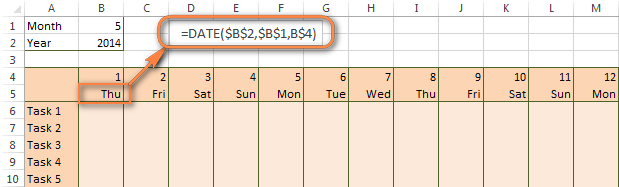Tip. If you want to display only the days of the week like you see in the image above, select the cells with the formula (row 5 in our case), right-click and choose Format Cells…> Number > Custom. From the drop-down list under Type, select either dddd or ddd to show full day names or abbreviated names, respectively.

Your Excel calendar is almost done, and you only need to change the color of weekends. Naturally, you are not going to color the cells manually. We'll have Excel format the weekends automatically by creating a conditional formatting rule based on the WEEKDAY formula.

1. You start by selecting your Excel calendar where you want to shade the weekends. In our case, it is the range \$B\$4:\$AE\$10. Be sure to start the selection with the 1st date column - Colum B in this example.
2. On the Home tab, click Conditional Formatting menu > New Rule.
3. Create a new formatting rule based on a formula, as explianed in How to create Excel conditional formatting rules with a formula.
4. In the "Format values where this formula is true" box, enter the following WEEKDAY formula that will determine which cells are Saturdays and Sundays: `=WEEKDAY(B\$5,2)>5`
5. Click the Format… button and set up your custom format by switching between the Font, Border and Fill tabs and playing with different formatting options. When done, click the OK button to preview the rule.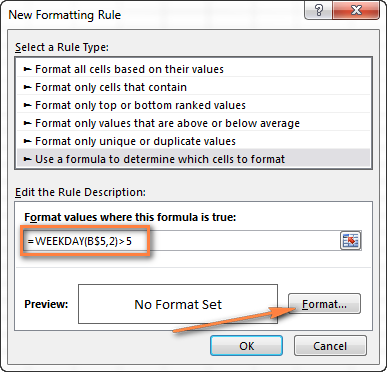Now, let me briefly explain the `WEEKDAY(serial_number,[return_type])` formula so that you can quickly adjust it for your own spreadsheets.

• The `serial_number` parameter represents the date you are trying to find. You enter a reference to your first cell with a date, B\$5 in our case.
• The `[return_type]` parameter determines the week type (square brackets imply it is optional). You enter 2 as the return type for a week starting from Monday (1) through Sunday (7). You can find the full list of available return types here.
• Finally, you write >5 to highlight only Saturdays (6) and Sundays (7).

The screenshot below demonstrates the result in Excel 2013 - the weekends are highlighted in the reddish colour.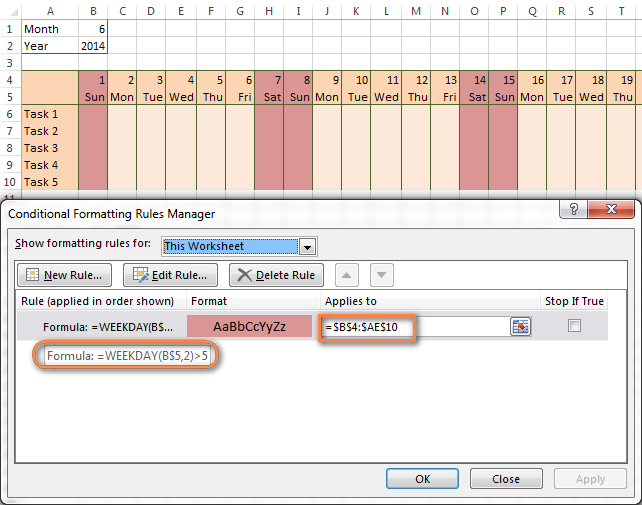Tips:

• If you have non-standard weekends in your company, e.g. Fridays and Saturdays, then you would need to tweak the formula so that it starts counting from Sunday (1) and highlight days 6 (Friday) and 7 (Saturday) - `WEEKDAY(B\$5,1)>5`.
• If you are creating a horizontal (landscape) calendar, use a relative column (without \$) and absolute row (with \$) in a cell reference because you should lock the reference of the row - in the above example it is row 5, so we entered B\$5. But if you are designing a calendar in vertical orientation, you should do the opposite, i.e. use an absolute column and relative row, e.g. \$B5 as you can see in the screenshot below: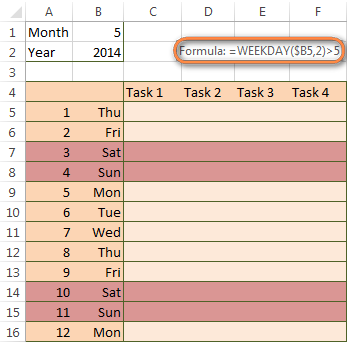### How to highlight holidays in Excel

To improve your Excel calendar further, you can shade public holidays as well. To do that, you will need to list the holidays you want to highlight in the same or some other spreadsheet.

For example, I've added the following holidays in column A (\$A\$14:\$A\$17). Of course, not all of them are real public holidays, but they will do for demonstration purposes : )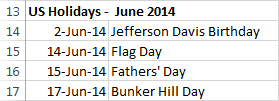Again, you open Conditional Formatting > New Rule. In the case of holidays, you are going to use either MATCH or COUNTIF function:

• `=COUNTIF(\$A\$14:\$A\$17,B\$5)>0`
• `=MATCH(B\$5,\$A\$14:\$A\$17,0)`
Note. If you have chosen a different color for holidays, you need to move the public holiday rule to the top of the rules list via Conditional Formatting > Manage Rules…

The following image shows the result in Excel 2013: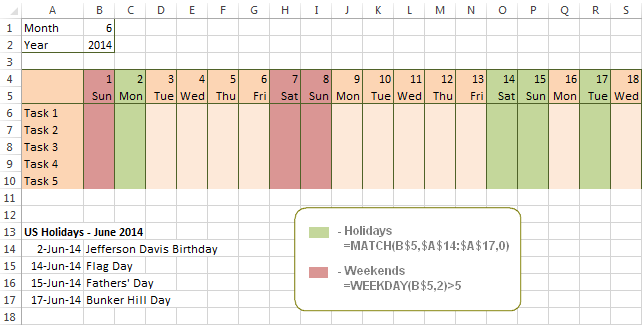### Conditionally format a cell when a value is changed to a date

It's not a big problem to conditionally format a cell when a date is added to that cell or any other cell in the same row as long as no other value type is allowed. In this case, you could simply use a formula to highlight non-blanks, as described in Excel conditional formulas for blanks and non-blanks. But what if those cells already have some values, e.g. text, and you want to change the background color when text is changed to a date?

The task may sound a bit intricate, but the solution is very simple.

1. First off, you need to determine the format code of your date. Here are just a few examples:
• D1: dd-mmm-yy or d-mmm-yy
• D2: dd-mmm or d-mmm
• D3: mmm-yy
• D4: mm/dd/yy or m/d/yy or m/d/yy h:mm

You can find the complete list of date codes in this article.

2. Select a column where you want to change the color of cells or the entire table in case you want to highlight rows.
3. And now create a conditional formatting rule using a formula similar to this one: `=CELL("format",\$A2)="D1"`. In the formula, A is the column with dates and D1 is the date format.

If your table contains dates in 2 or more formats, then use the OR operator, e.g. `=OR(cell("format", \$A2)="D1", cell("format",\$A2)="D2", cell("format", \$A2)="D3")`

The screenshot below demonstrates the result of such conditional formatting rule for dates.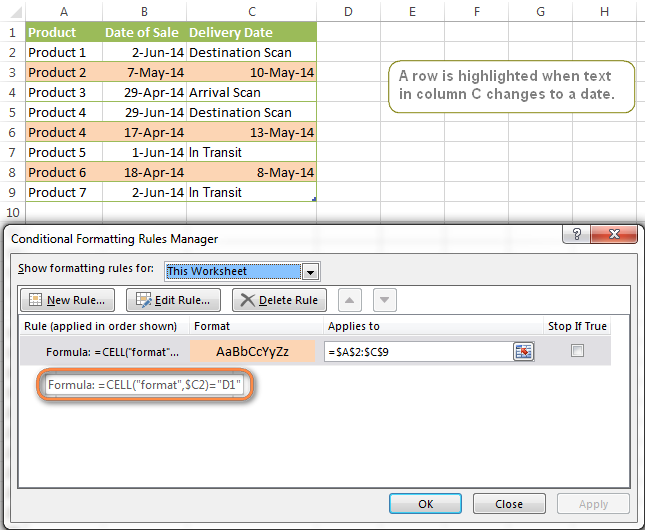### How to highlight rows based on a certain date in a certain column

Suppose, you have a large Excel spreadsheet that contains two date columns (B and C). You want to highlight every row that has a certain date, say 13-May-14, in column C.

To apply Excel conditional formatting to a certain date, you need to find its numerical value first. As you probably know, Microsoft Excel stores dates as sequential serial numbers, starting from January 1, 1900. So, 1-Jan-1900 is stored as 1, 2-Jan-1900 is stored as 2… and 13-May-14 as 41772.

To find the date's number, right-click the cell, select Format Cells > Number and choose the General format. Write down the number you see and click Cancel because you do not really want to change the date's format.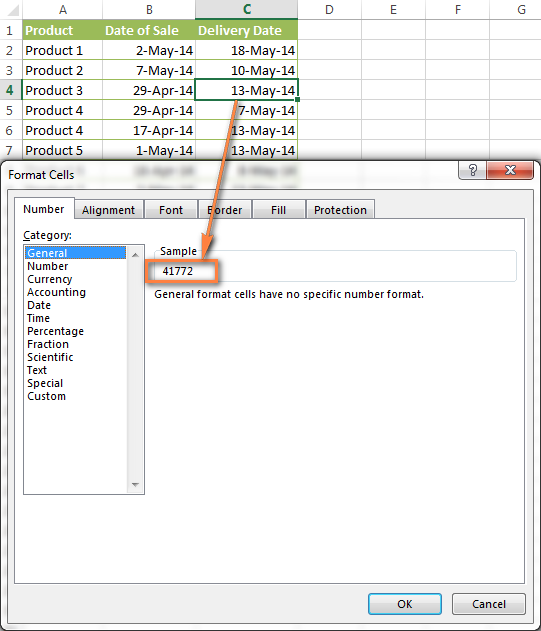That was actually the major part of the work and now you only need to create a conditional formatting rule for the entire table with this very simple formula: `=\$C2=41772`. The formula implies that your table has headers and row 2 is your first row with data.

An alternative way is to use the DATEVALUE formula that converts the date to the number format is which it is stored, e.g. `=\$C2=DATEVALUE("5/13/2014")`

Whichever formula you use, it will have the same effect: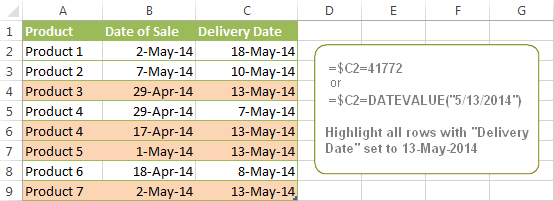### Conditionally format dates in Excel based on the current date

As you probably know Microsoft Excel provides the `TODAY()` functions for various calculations based on the current date. Here are just a few examples of how you can use it to conditionally format dates in Excel.

#### Example 1. Highlight dates equal to, greater than or less than today

To conditionally format cells or entire rows based on today's date, you use the TODAY function as follows:

Equal to today: `=\$B2=TODAY()`

Greater than today: `=\$B2>TODAY()`

Less than today: `=\$B2<TODAY()`

The screenshot below demonstrates the above rules in action. Please note, at the moment of writing TODAY was 12-Jun-2014.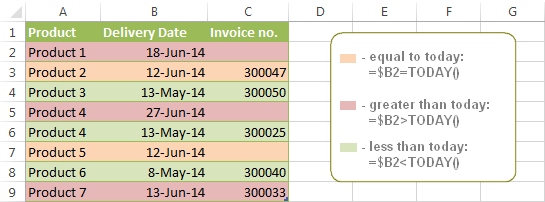#### Example 2. Conditionally format dates in Excel based on several conditions

In a similar fashion, you can use the TODAY function in combination with other Excel functions to handle more complex scenarios. For example, you may want your Excel conditional formatting date formula to color the Invoice column when the Delivery Date is equal to or greater than today BUT you want the formatting to disappear when you enter the invoice number.

For this task, you would need an additional column with the following formula (where E is your Delivery column and F the Invoice column):

`=IF(E2>=TODAY(),IF(F2="", 1, 0), 0)`

If the delivery date is greater than or equal to the current date and there is no number in the Invoice column, the formula returns 1, otherwise it's 0.

After that you create a simple conditional formatting rule for the Invoice column with the formula `=\$G2=1` where G is your additional column. Of course, you will be able to hide this column later.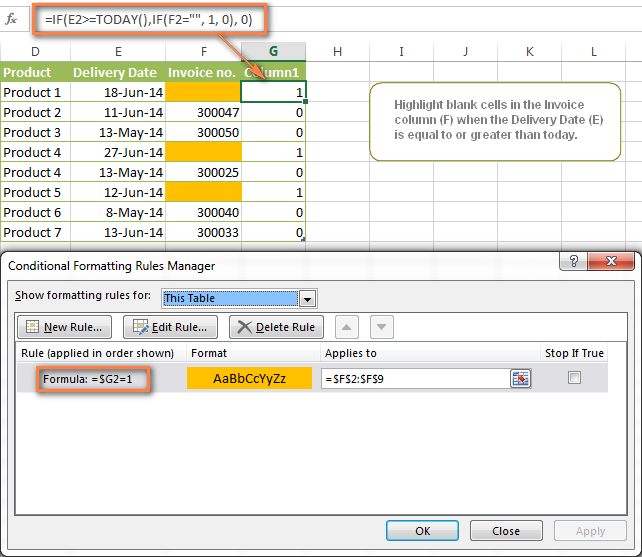#### Example 3. Highlight upcoming dates and delays

Suppose you have a project schedule in Excel that lists tasks, their start dates and durations. What you want is to have the end date for each task calculated automatically. An additional challenge is that the formula should also consider the weekends. For example, if the starting date is 13-Jun-2014 and the number of days of work (Duration) is 2, the ending date should come as 17-Jun-2014, because 14-Jun and 15-Jun are Saturday and Sunday.

To do this, we will use the `WORKDAY.INTL(start_date,days,[weekend],[holidays])` function, more precisely `=WORKDAY.INTL(B2,C2,1)`.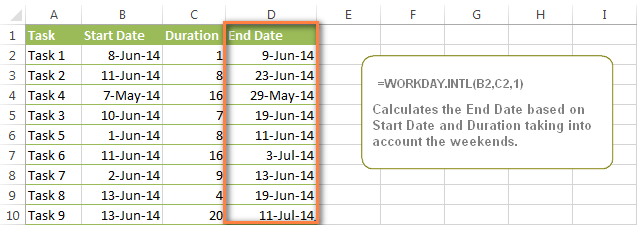In the formula, we enter 1 as the 3rd parameter since it indicates Saturday and Sunday as holidays. You can use another value if your weekends are different, say, Fri and Sat. The full list of the weekend values is available here. Optionally, you can also use the 4th parameter [holidays], which is a set of dates (range of cells) that should be excluded from the working day calendar.

And finally, you may want to highlight rows depending on how far away the deadline is. For example, the conditional formatting rules based on the following 2 formulas highlight upcoming and recent end dates, respectively:

• `=AND(\$D2-TODAY()>=0,\$D2-TODAY()<=7)` - highlight all rows where the End Date (column D) is within the next 7 days. This formula is really handy when it comes to tracking upcoming expiration dates or payments.
• `=AND(TODAY()-\$D2>=0,TODAY()-\$D2<=7)` - highlight all rows where the End Date (column D) is within the last 7 days. You can use this formula to track the latest overdue payments and other delays.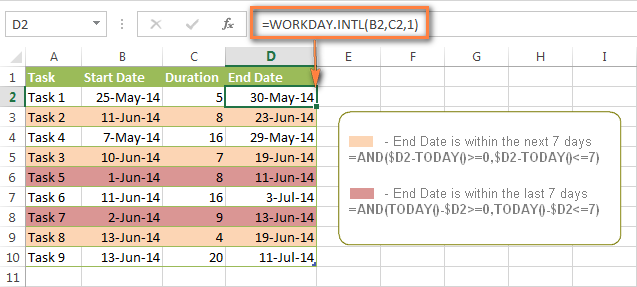Here are a few more formula examples that can be applied to the table above:

`=\$D2<TODAY()` - highlights all passed dates (i.e. dates less than the current date). Can be used to format expired subscriptions, overdue payments etc.

`=\$D2>TODAY()` - highlights all future dates (i.e. dates greater than the current date). You can use it to highlight upcoming events.

Of course, there can be infinite variations of the above formulas, depending on your particular task. For instance:

`=\$D2-TODAY()>=6` - highlights dates that occur in 6 or more days.

`=\$D2=TODAY()-14` - highlights dates occurring exactly 2 weeks ago.

### How to highlight dates within a date range

If you have a long list of dates in your worksheet, you may also want to highlight the cells or rows that fall within a certain date range, i.e. highlight all dates that are between two given dates.

You can fulfil this task using the TODAY() function again. You will just have to construct a little bit more elaborate formulas as demonstrated in the examples below.

Formulas to highlight past dates

• More than 30 days ago: `=TODAY()-\$A2>30`
• From 30 to 15 days ago, inclusive: `=AND(TODAY()-\$A2>=15, TODAY()-\$A2<=30)`
• Less than 15 days ago: `=AND(TODAY()-\$A2>=1, TODAY()-\$A2<15)`

The current date and any future dates are not colored.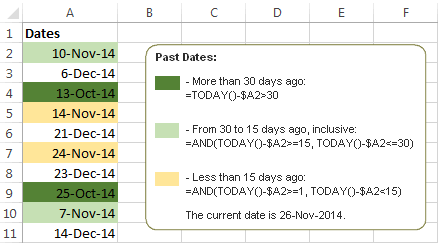Formulas to highlight future dates

• Will occur in more than 30 days from now: `=\$A2-TODAY()>30`
• In 30 to 15 days, inclusive: `=AND(\$A2-TODAY()>=15, \$A2-TODAY()<=30)`
• In less than 15 days: `=AND(\$A2-TODAY()>=1, \$A2-TODAY()<15)`

The current date and any past dates are not colored.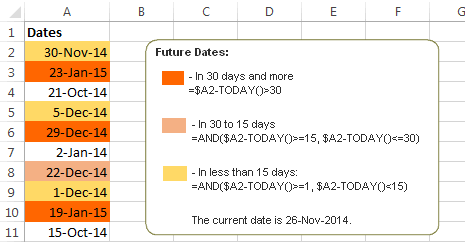### How to shade gaps and time intervals

In this last example, we are going to utilize yet another Excel date function - `DATEDIF(start_date, end_date, interval)`. This function calculates the difference between two dates based on the specified interval. It differs from all other functions we've discussed in this tutorial in the way that it lets you ignore months or years and calculate the difference only between days or months, whichever you choose.

Don't see how this could work for you? Think about it in another way… Suppose you have a list of birthdays of your family members and friends. Would you like to know how many days there are until their next birthday? Moreover, how many days exactly are left until your wedding anniversary and other events you wouldn't want to miss? Easily!

The formula you need is this (where A is your Date column):

`=DATEDIF(TODAY(), DATE((YEAR(TODAY())+1), MONTH(\$A2), DAY(\$A2)), "yd")`

The "yd" interval type at the end of the formula is used to ignore years and calculate the difference between the days only. For the full list of available interval types, look here.

Tip. If you happen to forget or misplace that complex formula, you can use this simple one instead: `=365-DATEDIF(\$A2,TODAY(),"yd")`. It produces exactly the same results, just remember to replace 365 with 366 in leap years : )

And now let's create an Excel conditional formatting rule to shade different gaps in different colors. In this case, it makes more sense to utilize Excel Color Scales (Conditional formatting > Color Scales) rather than create a separate rule for each period.

The screenshot below demonstrates the result in Excel - a gradient 3-color scale with tints from green to red through yellow.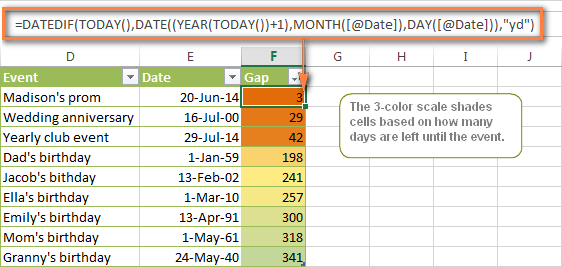#### "Days Until Next Birthday" Excel Web App

We have created this Excel Web App to show you the above formula in action. Just enter your events in 1st column and change the corresponding dates in the 2nd column to experiment with the result.War in Ukraine. Here's what Ablebits is doing to make sure our team and projects are safe.